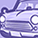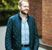# AURIX™ Forum DiscussionsLevel 1
Level 1

# TC277X DSP Function & Fixed point implementation

Hello everyone,

I need write code fixed point in tc277x controller.

How to perform arithmetic operation and Trigonometric function implementation in fixed point?.

Regards,
Moorthi M
2 RepliesEmployee
Employee# Re: TC277X DSP Function & Fixed point implementation

Fixed point means in general to abstract fractional numbers into signed integer arithmetic and not using floating-point.

Depending on the resolution needed you would use either Q1.15 or Q1.31.
Technically, both are still signed 16bit or 32bit numbers. But they are representing numbers between -1 to 0.999999999.

Example you want to multiply a signed ADC value of "8790" with a fractional number of -0.0089.

First -> Multiply -0.0089 with either 2^15 or 2^31 depending on the needed resolution which would be -292 in Q1.15 format or -19112604 in Q1.31 format.

Second -> Multiply your ADC Value of 8790 with -292 if using q1.15 -> leading to a result of -2566680

Third -> Apply an arithmetic right-shift of either 15 or 31 depending on the used Q1.xx format. -2566680>>15 = ~ -78.3

If you need bigger numerical ranges than -1 to +1, you could also use Q2.14 / Q2.30 or even Q3.13 or Q3.29 format and so on. The general concept stays the same.

If you have to multiply 8790 with a value of -1.7898, then you would use the q2.14 format. -1.7898*(2^14)= -29324.
Afterwards multiply 8790 with -29324 = -257757960
Apply right shift of 14 -> -257757960>>14 = ~-15732.3

If you need to implement trigonometric functions in fixed-point, I just found this on the web which looks quite good - but I haven't tried it out:
https://www.nullhardware.com/blog/fixed-point-sine-and-cosine-for-embedded-systems/#:~:text=The%20do....Employee
Employee# Re: TC277X DSP Function & Fixed point implementation

Sorry - totally forgot to mention that there is also the dsp-library available for Aurix in myICP.
There is a lot of stuff which is optimized for the TriCore platform.